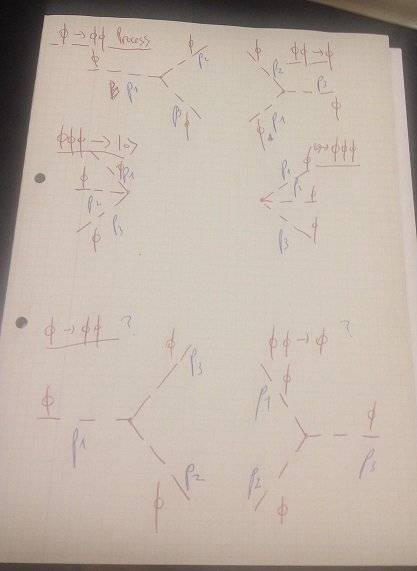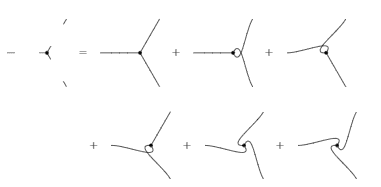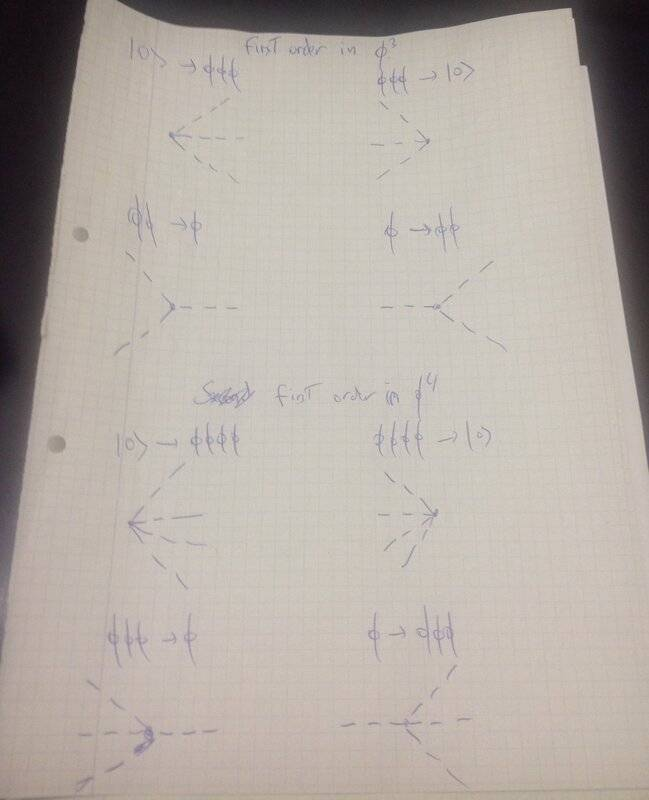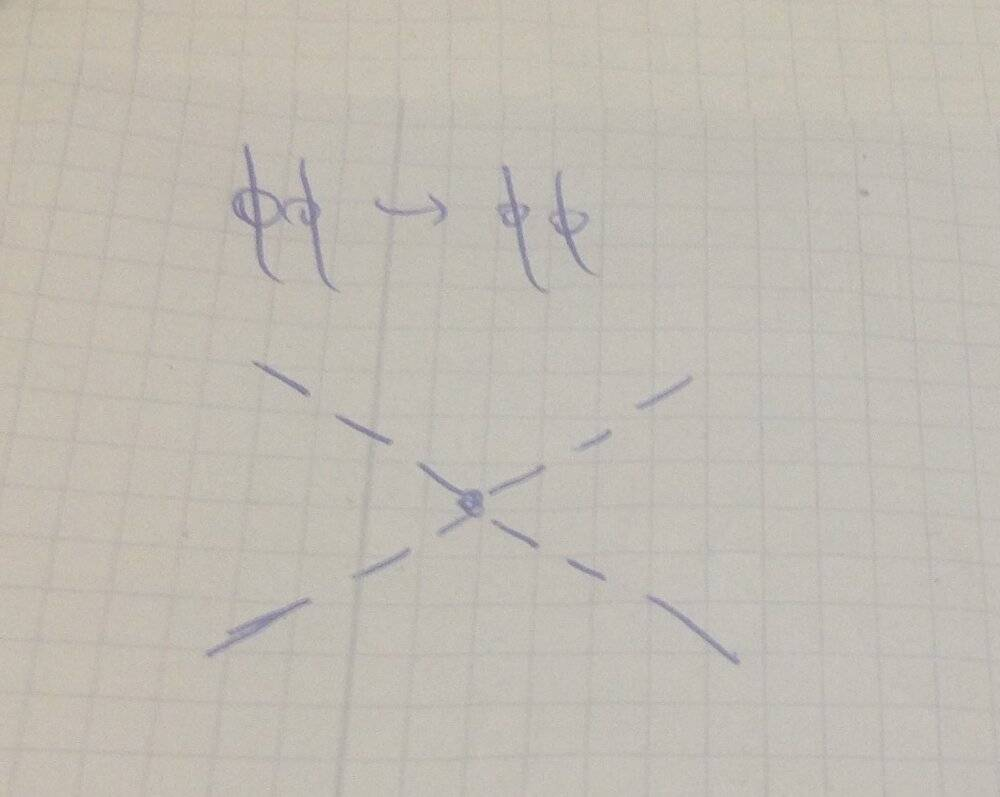# Feynman Rules on ##\phi^3, \phi^4## theory

Homework Helper
Yes

JD_PM
Then we get

\begin{align*}
&\langle 0| b(\vec p_2)b(\vec p_3)b^{\dagger}(\vec k_2) b^{\dagger}(\vec k_3)b(\vec k_1)b^{\dagger}(\vec p_1)|0\rangle = \langle 0| b(\vec p_2)[b(\vec p_3),b^{\dagger}(\vec k_3)]b^{\dagger}(\vec k_2)[b(\vec k_1),b^{\dagger}(\vec p_1)]|0\rangle \\
&= \delta_{\vec p_3, \vec k_3} \delta_{\vec p_1, \vec k_1} \langle 0 | [b(\vec p_2), b^{\dagger}(\vec k_2)] |0\rangle \\
&= \delta_{\vec p_3, \vec k_3} \delta_{\vec p_1, \vec k_1}\delta_{\vec p_2, \vec k_2}
\end{align*}

Homework Helper
I agree on
\begin{align*} &\langle 0| b(\vec p_2)b(\vec p_3)b^{\dagger}(\vec k_2) b^{\dagger}(\vec k_3)b(\vec k_1)b^{\dagger}(\vec p_1)|0\rangle = \langle 0| b(\vec p_2)b(\vec p_3)b^{\dagger}(\vec k_3) b^{\dagger}(\vec k_2)[b(\vec k_1),b^{\dagger}(\vec p_1)]|0\rangle \\ &=\delta_{\vec p_1, \vec k_1} \langle 0 | b(\vec p_2)b(\vec{p}_3) b^\dagger (\vec{k}_3)b^{\dagger}(\vec k_2)|0\rangle \\ \end{align*}
But I still don't see how you get from here a ##\delta_{\vec p_3, \vec k_3}##

JD_PM
I am also using that ##[b^{\dagger}(\vec k_2), b^{\dagger}(\vec k_3)]=0##. Then I can form another commutation relation as shown in #37

Homework Helper
I don't see how. Could you elaborate a little bit more in how you do it?

JD_PM
Thank you for asking further! We have

\begin{align*}

&\langle 0| b(\vec p_2)b(\vec p_3)b^{\dagger}(\vec k_2) b^{\dagger}(\vec k_3)b(\vec k_1)b^{\dagger}(\vec p_1)|0\rangle = \langle 0| b(\vec p_2)b(\vec p_3)b^{\dagger}(\vec k_3) b^{\dagger}(\vec k_2)[b(\vec k_1),b^{\dagger}(\vec p_1)]|0\rangle \\

&=\delta_{\vec p_1, \vec k_1} \langle 0 | b(\vec p_2)b(\vec{p}_3) b^\dagger (\vec{k}_3)b^{\dagger}(\vec k_2)|0\rangle = \delta_{\vec p_1, \vec k_1} \langle 0 | b(\vec p_3)b(\vec{p}_2) b^\dagger (\vec{k}_3)b^{\dagger}(\vec k_2)|0\rangle\\

&= \delta_{\vec p_1, \vec k_1} \langle 0 | b(\vec p_3) b^\dagger (\vec{k}_3)b(\vec{p}_2)b^{\dagger}(\vec k_2)|0\rangle \\

&= \delta_{\vec p_1, \vec k_1} \langle 0 | [b(\vec p_3), b^\dagger (\vec{k}_3)][b(\vec{p}_2),b^{\dagger}(\vec k_2)]|0\rangle \\

&= \delta_{\vec p_3, \vec k_3} \delta_{\vec p_1, \vec k_1}\delta_{\vec p_2, \vec k_2}

\end{align*}

Where I've used

$$[b(\vec p_2),b(\vec{p}_3)]=0$$

$$[b (\vec{p}_2), b^{\dagger}(\vec k_3)]=0$$

I think the main confusion is with ##[b (\vec{p}_2), b^{\dagger}(\vec k_3)]=0##. I think that it indeed holds because we are not considering the same 'helicity' (maybe for mesons there is another term but the important issue is that we need the sublabels to be equal, otherwise the commutator vanishes). More examples are

$$[b (\vec{p}_1), b^{\dagger}(\vec k_3)]=0$$

$$[b (\vec{p}_1), b^{\dagger}(\vec k_2)]=0$$

To be precise, the commutator relation is (my bad, I forgot to include the 'helicity' back in #35)

\begin{equation*}

[b(\vec p_n), b^{\dagger}(\vec k_m)]=\delta_{\vec p, \vec k}\delta_{n, m}

\end{equation*}

Do you agree now?Homework Helper
Ok, no I don't agree, in Klein-Gordon fields, the creation and annihilation operators are completely defined by the momentum, there's no helicity involved and I disagree with all the commutators you have except for
$$[b(\vec p_2), b(\vec p_3)]=0$$.
The names of the momenta are completely arbitrary, we could have called ##\vec p_2## to the momentum of the initial particle, or ##\vec q##, and is even worse with the ##\vec k_i## since they are dummy labels, we could exchange ##\vec k_2## for ##\vec k_3## and then
$$[b (\vec{p}_2), b^{\dagger}(\vec k_3)]=0$$
transform into
$$[b(\vec{p}_2), b^\dagger(\vec{k}_2)]=0$$
and the hole calculation is zero.
Therefore
$$[b(\vec k), b^\dagger (\vec p)] = \delta_{\vec k \vec p}$$
independently, of course, of what is the name you use for the momentum.

•JD_PM
JD_PM
Oh so let's assume there is no helicity involved.

Then, indeed we get

\begin{align*}

&\langle 0| b(\vec p_2)b(\vec p_3)b^{\dagger}(\vec k_2) b^{\dagger}(\vec k_3)b(\vec k_1)b^{\dagger}(\vec p_1)|0\rangle = \langle 0| b(\vec p_2)b(\vec p_3)b^{\dagger}(\vec k_3) b^{\dagger}(\vec k_2)[b(\vec k_1),b^{\dagger}(\vec p_1)]|0\rangle \\

&=\delta_{\vec p_1, \vec k_1} \langle 0 | b(\vec p_2)b(\vec{p}_3) b^\dagger (\vec{k}_3)b^{\dagger}(\vec k_2)|0\rangle \\

\end{align*}

I see no way of reducing this further. Is there a way of doing it though?

Should I simply modify my computation in #29 at this point of am I missing something else?

Homework Helper
Of course, the computation in #29 you use these wrong commutation relations and therefore is not correct. To keep simplifying (well, simplify is not a well-defined word) but to write this expression to something you can work with you need to get rid of this expectation value. My best advice is: try to move all the creation operators to the left (or all the annihilation to the right). Of course, this cannot be done trivially, but using the fact that
$$AB = BA + [A,B]$$ and that all the commutators are c-numbers and therefore commute with all the operators and can go outside the vev, you can "simplify" a lot.

•JD_PM
JD_PM
Let's proceed then

\begin{align*}

&=\delta_{\vec p_1, \vec k_1} \langle 0 | b(\vec p_2)b(\vec{p}_3) b^\dagger (\vec{k}_3)b^{\dagger}(\vec k_2)|0\rangle = \delta_{\vec p_1, \vec k_1} \langle 0 | b(\vec p_2) \left( b^\dagger (\vec{k}_3)b(\vec{p}_3) +\delta_{\vec k_3, \vec p_3} \right) b^{\dagger}(\vec k_2)|0\rangle\\

&= \delta_{\vec p_1, \vec k_1} \langle 0 | [b(\vec p_2), b^\dagger (\vec{k}_3)][b(\vec{p}_3), b^{\dagger}(\vec k_2)]|0\rangle + \delta_{\vec p_1, \vec k_1}\delta_{\vec k_3, \vec p_3} \langle 0 | [b(\vec p_2),b^{\dagger}(\vec k_2)] |0\rangle\\

&= \delta_{\vec p_1, \vec k_1}\delta_{\vec p_2, \vec k_3}\delta_{\vec p_3, \vec k_2}+\delta_{\vec p_1, \vec k_1}\delta_{\vec k_3, \vec p_3}\delta_{\vec k_2, \vec p_2}

\end{align*}

So I indeed missed a term! Now it should be correct.

If you agree with such computation I'll go and fix #29

Homework Helper
Ok, now perfect

•JD_PM
JD_PM
I think I got it!😍

\begin{align*}
&-3 i \frac{\lambda_3}{3!}\int d^4 x \langle f| \phi^- \phi^- \phi^+ (x) |i \rangle = \\
&=-3i \frac{\lambda_3}{3!}\sum_{\vec k_2}\sum_{\vec k_3}\sum_{\vec k_1}\left(\frac{1}{2V \omega_{\vec k_2}}\right)^{1/2}\left(\frac{1}{2V \omega_{\vec k_3}}\right)^{1/2}\left(\frac{1}{2V \omega_{\vec k_1}}\right)^{1/2} \times \\
&\times \langle 0| b(\vec p_2)b(\vec p_3)b^{\dagger}(\vec k_2) b^{\dagger}(\vec k_3)b(\vec k_1)b^{\dagger}(\vec p_1)|0\rangle \int d^4 x \exp\left(-ix\cdot(k_1-k_2-k_3)\right) \\
&=-3i \frac{\lambda_3}{3!}\sum_{\vec k_2}\sum_{\vec k_3}\sum_{\vec k_1}\left(\frac{1}{2V \omega_{\vec k_2}}\right)^{1/2}\left(\frac{1}{2V \omega_{\vec k_3}}\right)^{1/2}\left(\frac{1}{2V \omega_{\vec k_1}}\right)^{1/2} \times \\
&\times \left(\delta_{\vec p_1, \vec k_1}\delta_{\vec p_2, \vec k_3}\delta_{\vec p_3, \vec k_2}+\delta_{\vec p_1, \vec k_1}\delta_{\vec k_3, \vec p_3}\delta_{\vec k_2, \vec p_2}\right) (2\pi)^4 \delta^{(4)} (k_1-k_2-k_3) \\
&= -2\times 3i \frac{\lambda_3}{3!}\left(\frac{1}{2V \omega_{\vec p_1}}\right)^{1/2}\left(\frac{1}{2V \omega_{\vec p_2}}\right)^{1/2}\left(\frac{1}{2V \omega_{\vec p_3}}\right)^{1/2} (2\pi)^4 \delta^{(4)} (p_1-p_2-p_3)
\end{align*}

So the Feynman amplitude is ##\mathcal{M}=-i\lambda_3##

Mmm now I am a bit confused. If the computation is correct, does this mean that the interaction vertex for the ##\phi^3## term is ##-i\lambda_3##? I was expecting ##-i\lambda_3/3!##, as vanhees71 suggested at #2

Homework Helper
Well, that depends on how you define the Feynman rule for the vertex, ##\lambda##. If you want to consider all the different ways to connect the external lines to the vertex, as different diagrams, then the computation we have done will be proportional to ##3!\lambda## (because you will have 3! different diagrams). And, therefore, you will obtain that the Feynman rule is $$\lambda = \frac{-i\lambda_3}{3!}$$if otherwise, you want to consider all the possible ways of connecting the lines as the same diagram, then the computation is proportional to ##\lambda## and you have $$\lambda = -i\lambda_3$$personally I have always seen this second one, but there's nothing wrong with the other.

Also, you haven't proved that the term proportional to ##\lambda_4## vanish, so, strictly speaking, there could be still a contribution proportional to ##\lambda_4##.

Then the computation for the vertex for the other interaction is completely analogous, I recommend you to do it.

•JD_PM
JD_PM
Well, that depends on how you define the Feynman rule for the vertex, ##\lambda##. If you want to consider all the different ways to connect the external lines to the vertex, as different diagrams, then the computation we have done will be proportional to ##3!\lambda## (because you will have 3! different diagrams). And, therefore, you will obtain that the Feynman rule is $$\lambda = \frac{-i\lambda_3}{3!}$$if otherwise, you want to consider all the possible ways of connecting the lines as the same diagram, then the computation is proportional to ##\lambda## and you have $$\lambda = -i\lambda_3$$personally I have always seen this second one, but there's nothing wrong with the other.

I have been thought the first one, do you mind if we stick to it?

Well, that depends on how you define the Feynman rule for the vertex, ##\lambda##. If you want to consider all the different ways to connect the external lines to the vertex, as different diagrams, then the computation we have done will be proportional to ##3!\lambda## (because you will have 3! different diagrams).

I've been thinking about this but I do not fully see the 3!=6 possible diagrams. The Feynman diagrams I get areThe first four are OK but what about the last two?

But how can we be sure that there are 3! possible diagrams, before actually check it out explicitly? Is Wick's theorem a way to check that out?

Homework Helper
Nop. you are drawing diagrams for different processes, that's not what I'm talking about, if you want to take the first option there are 6 diagrams to draw for the ##\phi \to 2\phi## process. You have 3 external lines (representing the 3 particles at infinity) and 3 legs of the vertex to connect. Therefore 6 possibilities (3 for the first pair, 2 for the second, and the last one is immediately determined).

•JD_PM
JD_PM
You have 3 external lines (representing the 3 particles at infinity) and 3 legs of the vertex to connect. Therefore 6 possibilities (3 for the first pair, 2 for the second, and the last one is immediately determined).

OK I get we have 3 external lines but what do you mean by 'legs of the vertex' ?

Homework Helper
Let's see if I can light your way, in the picture, I draw a first diagram, where you can see the three external lines (the outer ones) and the three legs of the vertex (the three lines touching the point). What you need is to pair these lines (one external with one leg of the vertex), there are six ways to do it, which I have drawn after the ##=## sign.Note I am using the orientation of the external and internal lines as a way to distinguish them, but in reality, we can adjust these directions to make the six diagrams look exactly the same as the first one.

•JD_PM
JD_PM
Sorry but I am afraid I got lost.

what kind of rules are you following to draw the internal lines? I do not understand why you are allowed to curve them (I was only thinking in terms of straight lines)

Homework Helper
I'm just connecting the external lines with the legs of the vertex with lines, as always in Feynman diagrams, curved lines or straight lines make no difference, is just a way to make them look nicer. If I write only straight lines then all the pictures look the same and there's no way to see why there are 6 and only 6.
There's no secret, just draw on a paper the external lines and the legs of the vertex (i.e. draw on a paper the picture on the left of the ##=##) and then connect them by lines...
In any case, if you are not used to this kind of pictures, is better to forget about the 3!, and take
$$\lambda = -i\lambda_3$$as your Feynman rule (which is what everyone does), and then you will need to draw only one diagram.
Although I must say that this kind of pictures are really useful to determine symmetry factors.

•JD_PM
JD_PM
OK thank you.

Before showing that the term proportional to ##\lambda_4## vanishes, please let me do an overall checking to see if I understand the whole process.

In first order, we have checked in detail the ##\phi \to \phi \phi## process and shown that its Feynman amplitude is ##\mathcal{M}= -i\lambda_3##. Let's look at the other first order terms

For ##|0\rangle \to \phi \phi \phi##, ##\phi \phi \phi \to |0\rangle## and ##\phi \phi \to \phi## I get the exact same Feynman amplitude i.e. ##\mathcal{M}= -i\lambda_3##; What is this telling us?

Homework Helper
Well, this tells us that the Feynman rule associated with the vertex is independent of the details on how this vertex is connected to the rest of the diagram. For each vertex, you multiply by the same factor, i.e. ##-i\lambda_3##.
If this were not true then you couldn't have such a general rule, you should have different vertex factor for different cases, fortunately, it's not the case.

•JD_PM
JD_PM
Just to check my basic understanding of Feynman diagrams; these are the basic (i.e. only first order) processes of our theory (in a very schematic way)Homework Helper
Correct

•JD_PM
JD_PM
$$S = \langle \phi\phi | \phi \rangle + i \frac{\lambda_3}{3!} \int d^4 x \langle \phi\phi| :\phi(x) \phi(x) \phi (x): |\phi\rangle + i\frac{\lambda_4}{4!} \int d^4 x \langle \phi\phi | :\phi(x) \phi(x) \phi(x) \phi (x) : | \phi \rangle$$
Now show that the first and last terms vanish and then, from all the terms arising from splitting ##\phi = \phi^+ + \phi^-## look which ones survive.

Let's show that the last term vanishes!Let's focus on the matrix element ##\langle f| :\phi(x) \phi(x) \phi(x) \phi (x) : |i \rangle##; we have

$$\langle 0|b(\vec p_2) b(\vec p_3) b(\vec k_2) b(\vec k_3) \phi (x) b(\vec k_1) b^{\dagger}(\vec p_1) |0 \rangle$$

So we see there's a 'free variable'; that's because we are only dealing with 3 fields (i.e. we are studying the process ##\phi \to \phi\phi##) while there are 4 nestled. Then we have

\begin{align*}
&\langle 0|b(\vec p_2) b(\vec p_3) b^{\dagger}(\vec k_2) b^{\dagger}(\vec k_3) (b(\vec k)+b^{\dagger}(\vec k)) b(\vec k_1) b^{\dagger}(\vec p_1) |0 \rangle \\
&= \langle 0|b(\vec p_2) b(\vec p_3) b^{\dagger}(\vec k_2) b^{\dagger}(\vec k_3) b(\vec k) b(\vec k_1) b^{\dagger}(\vec p_1) |0 \rangle + \langle 0|b(\vec p_2) b(\vec p_3) b^{\dagger}(\vec k_2) b^{\dagger}(\vec k_3) b^{\dagger}(\vec k) b(\vec k_1) b^{\dagger}(\vec p_1) |0 \rangle
\end{align*}

In the ##\langle 0|b(\vec p_2) b(\vec p_3) b^{\dagger}(\vec k_2) b^{\dagger}(\vec k_3) b(\vec k) b(\vec k_1) b^{\dagger}(\vec p_1) |0 \rangle## term we observe that ##b(\vec k)## will hit ##|0\rangle## so this term will vanish. Besides, in the ##\langle 0|b(\vec p_2) b(\vec p_3) b^{\dagger}(\vec k_2) b^{\dagger}(\vec k_3) b^{\dagger}(\vec k) b(\vec k_1) b^{\dagger}(\vec p_1) |0 \rangle## term we observe that ##b^{\dagger}(\vec k)## will hit ##\langle 0 |## so this term will vanish.

Thus we indeed get

$$i\frac{\lambda_4}{4!} \int d^4 x \langle \phi\phi | :\phi(x) \phi(x) \phi(x) \phi (x) : | \phi \rangle =0$$

PS: @Gaussian97 again, another really fun and useful discussion! Again, I hope to come across you very soon!Homework Helper
$$\langle 0|b(\vec p_2) b(\vec p_3) b(\vec k_2) b(\vec k_3) \phi (x) b(\vec k_1) b^{\dagger}(\vec p_1) |0 \rangle$$
Well, in fact you should study
$$\langle 0|b(\vec p_2) b(\vec p_3) \phi(x)\phi(x) \phi (x) \phi(x) b^{\dagger}(\vec p_1) |0 \rangle$$
but yes, the reason is very obvious you can always move an anihilation operator to the right or a creation to the left, so all the possible terms will vanish.
Indeed, it's a good exercise to prove that any expectation value of the form
$$\left< 0\right|\prod_{i=0}^n\prod_{j=1}^{m_i} b^{(i)}(k_{ij})\left|0\right>,\quad \text{where}\quad b^{(i)}=\begin{cases}b& \text{ for } i = \dot{2}\\ b^{\dagger}& \text{ for } i \neq \dot{2}\end{cases}$$
and where ##n\neq \dot{2}## (if ##n## is even then the expresion is trivial since there are anihilation operators acting on the vacuum state). This is always zero unless
$$\sum_{i=0}^n(-1)^{i}m_i = 0$$
Then the case
$$\langle 0|b(\vec p_2) b(\vec p_3) b^{\dagger}(\vec k_2) b^{\dagger}(\vec k_3) b(\vec k) b(\vec k_1) b^{\dagger}(\vec p_1) |0 \rangle$$
is just given by ##n=3, m_0=2, m_1=2, m_2=2, m_3=1## and therefore ##m_0-m_1+m_2-m_3=1\neq 0## so that expression must be zero, and similarly
$$\langle 0|b(\vec p_2) b(\vec p_3) b^{\dagger}(\vec k_2) b^{\dagger}(\vec k_3) b^{\dagger}(\vec k) b(\vec k_1) b^{\dagger}(\vec p_1) |0 \rangle$$
is the case for ##n=3, m_0=2, m_1=3, m_2=1, m_3=1## so ##m_0-m_1+m_2-m_3=-1## so obviously is also zero.
You can use this formula to prove that all the other terms in the expansion of ##\phi^4## vanish.

•JD_PM